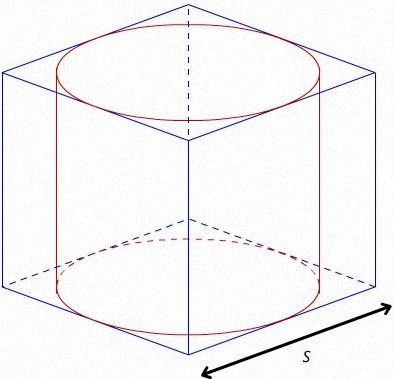SEARCH HOMEMath Central Quandaries & QueriesQuestion from Sonia, a student: A cylinder has the same height and diameter as the sides of a cube use 3.14~ which has the greater volumeHi Sonia,

If $S$ is the length of a side of the cube then you can find its volume as $S^{3}.$ The radius of the base of the circle is $\large \frac{S}{2}$ and the volume of a cylinder is the area of the base times the height. You don't need to do any calculations however to answer the question. Just draw a diagram.Image from Open Study

PennyMath Central is supported by the University of Regina and the Imperial Oil Foundation.